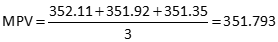## 1. Introduction

The smaller the expected random error in a measurement, the higher its quality. What happens when we combine different quality measurements?

Let's say three crews measured the same distance using different equipment. Their results are:

 Method Distance Precision Stadia 352.11 1/400 Steel tape 351.92 1/1000 Total Station Inst (TSI) 351.35 1/10,000

What's the distance? We could use a simple average of the three distances:Even though, by definition, the MPV is the value which minimizes the sum of the squares of the residuals, is it the best representation of the distance? Although they are of mixed quality, each measurement has the same effect on the MPV. From the precisions, it's obvious the TSI measurement is better that the others, We should allow it greater influence on the MPV. We do this by assigning weights based on measurement quality.

The weighted mean is computed using Equation E-1Equation E-1 meas: Measurement w: Weight

A measurement's weight is inversely proportional to its error - the smaller the error, the greater the weight. Assuming measurements mistakes and systematic errors have been compensated, precision can be used as an accuracy surrogate. Taking the inverse of each precision can lead to some pretty big numbers. To keep them reasonably small we can divide each by the lowest weight.

 Method Distance Precision Weight Weight Stadia 352.11 1/400 400 1 Steel tape 351.92 1/1000 1000 2.5 Total Station Inst (TSI) 351.35 1/10,000 10,000 25

Based on weights, the MPV isThe MPV is "pulled" toward the TSI measurement since it is the highest quality.

The simple average is sometimes referred to as the unweighted mean. It can also be considered to have unit weighting, that is, the weight of each measurement is 1.